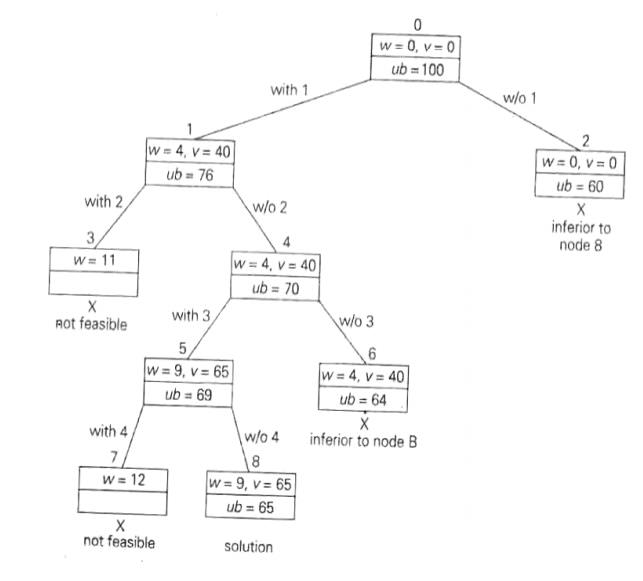Home | | User Interface Design | Branch and Bound - Knapsack Problem

# Branch and Bound - Knapsack Problem

Given n items of known weights wi and values vi, i = 1,2,..., n, and a knapsack of capacity W, find the most valuable subset of the items that fit in the knapsack.

KNAPSACK PROBLEM

Illustration

Given n items of known weights wi and values vi, i = 1,2,..., n, and a knapsack of capacity W, find the most valuable subset of the items that fit in the knapsack. It is convenient to order the items of a given instance in descending order by their value-to-weight ratios. Then the first item gives the best payoff per weight unit and the last one gives the worst payoff per weight unit, with ties resolved arbitrarily:It is natural to structure the state-space tree for this problem as a binary tree constructed as follows (following figure).

Each node on the ith level of this tree, 0 Ōēż i Ōēż n, represents all the subsets of n items that include a particular selection made from the first i ordered items. This particular selection is uniquely determined by a path from the root to the node: a branch going to the left indicates the inclusion of the next item while the branch going to the right indicates its exclusion.

We record the total weight w and the total value v of this selection in the node, along with some upper bound ub on the value of any subset that can be obtained by adding zero or more items to this selection.

A simple way to compute the upper bound ub is to add to v, the total value of the items already selected, the product of the remaining capacity of the knapsack W - w and the best per unit payoff among the remaining items, which is vi+1/wi+1 :As a specific example, let us apply the branch-and-bound algorithm to die same instance of

the knapsack problem , At the root of the state-space tree (in the following figure), no items have been selected as yet. Hence, both the total weight of the items already selected w and their total value v are equal to 0.

The value of the upper bound computed by formula (ub=v+(W-w)(vi+1/wi+1) is \$100. Node 1, the left child of the root, represents the subsets that include item, 1.

The total weight and value of the items already included are 4 and \$40, respectively; the value of the upper bound is 40 + (10-4)*6 = \$76.Fig: State-space tree of the branch-and-bound algorithm for the instance of the knapsack problem

Node 2 represents the subsets that do not include item 1. Accordingly, w = 0, v= \$0, and ub=0+(10-0)*6=\$60.

Since node 1 has a larger upper bound than the upper bound of node 2, it is more promising for this maximization problem, and we branch from node 1 first. Its childrenŌĆönodes 3 and 4ŌĆörepresent subsets with item 1 and with and without item 2, respectively.

Since the total weight w of every subset represented by node 3 exceeds the knapsackŌĆśs capacity, node 3 can be terminated immediately. Node 4 has the same values of w and u as its parent; the upper bound ub is equal to 40 + (10-4)*5 = \$70.

Selecting node 4 over node 2 for the next branching, we get nodes 5 and 6 by respectively including and excluding item 3. The total weights and values as well as the upper bounds for these nodes are computed in the same way as for the preceding nodes.

Branching from node 5 yields node 7, represents no feasible solutions and node 8 that represents just a single subset {1, 3}.

The remaining live nodes 2 and 6 have smaller upper-bound values than the value of the solution represented by node 8. Hence, both can be terminated making the subset {1, 3} of node 8 the optimal solution to the problem.

Solving the knapsack problem by a branch-and-bound algorithm has a rather unusual characteristic.

Typically, internal nodes of a state-space tree do not define a point of the problemŌĆśs search space, because some of the solutionŌĆśs components remain undefined. For the knapsack problem, however, every node of the tree represents a subset of the items given.

We can use this fact to update the information about the best subset seen so far after generating each new node in the tree. If we did this for the instance investigated above, we could have terminated nodes 2 & 6 before node 8 was generated because they both are inferior to the subset of value \$65 of node 5.

Study Material, Lecturing Notes, Assignment, Reference, Wiki description explanation, brief detail
Analysis and Design of Algorithm : Traversals, Branch and Bound : Branch and Bound - Knapsack Problem |